﻿ Computer Vision for Estimating Cooper Density by Optical Microscope Images

### Computer Vision for Estimating Cooper Density by Optical Microscope Images

Majid Memarian SorkhabiOPEN ACCESSPEER-REVIEWED

## Computer Vision for Estimating Cooper Density by Optical Microscope Images

Majid Memarian SorkhabiInstructor, University Collage of Roshdiyeh, Tabriz, Iran

### Abstract

Computer vision is one of the innovative methods in structure analyzing. In this paper computer vision and digital image processing were used for predict area of copper particle in the mineral soil. Iran is one of the most important mineral producers in the word and ranked among 15 major mineral-rich countries. The overall purpose has been to develop skills and techniques on detection and quantification of mineralogy and microstructures by means of various optical microscopy techniques and to study important microstructure parameters. In this research three new techniques are introduced: in first technique: images were decomposed to red, green and blue images. Secondly continuous and discrete wavelet transform of soil images were calculated. Finally percentage of energy for each wavelet coefficient was calculated. Accuracy of this analyze is under ±7.60% error in copper area estimation. symlets is selected the best wavelet for mineral analyzing. The threshold of copper energy coefficient is calculated equal to 3.23x10-4 in optical microscope images.

### At a glance: Figures

12345
Prev Next

• Sorkhabi, Majid Memarian. "Computer Vision for Estimating Cooper Density by Optical Microscope Images." American Journal of Computing Research Repository 2.4 (2014): 61-65.
• Sorkhabi, M. M. (2014). Computer Vision for Estimating Cooper Density by Optical Microscope Images. American Journal of Computing Research Repository, 2(4), 61-65.
• Sorkhabi, Majid Memarian. "Computer Vision for Estimating Cooper Density by Optical Microscope Images." American Journal of Computing Research Repository 2, no. 4 (2014): 61-65.

 Import into BibTeX Import into EndNote Import into RefMan Import into RefWorks

### 1. Introduction

During recent decades the Iranian mining industry has undergone a marked shift from being an industry largely based on exploitation of copper ores. Close to %30 of Iran's investment has been made in the mining field in recent years.

The production and export value of industrial minerals have continued to increase considerably. The industrial mineral production is characterized both by the variety of minerals and mineral products and by the fact that Iran is the world’s major producer of certain minerals.

The growth in industrial mineral production exceeds the decline in metallic ores, and it is recognized that there is a considerable future growth potential in industrial mineral production in Iran. The value of industrial minerals is primarily created during the beneficiation and the production of special products, needing both competence and sophisticated technology.

In 2009 Iran produced 383,000 tons of copper. As of 2011, Iran had the world's 9th largest reserves of copper at 32.5 million tons .

All mineral processes treat particles and not chemical elements. The particles contain one or more minerals. To achieve high quality products meeting the different requirements from the customers, and with a minimum waste, a thorough and detailed knowledge concerning the occurrence of the minerals in raw materials, products and waste is needed.

For a long time in investigations of particularly the base metal sulphides and precious minerals, electron microscope image analyzers have been used to quantify the occurrence of the minerals. Special emphasis has been laid on determining the liberation properties of the economic minerals to avoid under or over grinding of the minerals for further separation. It is recognized that the behavior of particles in separation processes is a function of different properties of which the most important are:

-Textural properties (shape, size, grain boundaries, type of intergrowths (particle texture)).

-Physical properties of the minerals (surface tension, specific gravity, magnetic property etc.).

-Mineralogical parameters (mineral chemistry, minerals in particle).

Due to low accuracy and Precision of human eyes, using microscope for mentioned properties did not trustable for micron and submicron grain analyzing.

Because of unavoidable premium of computer vision, many studies have been accomplished to use computer vision in mining industry [2, 3]. Image processing is used for image quality improvement for conveniences in image interpret and computer perceptions for gaining Optimum features.

To quantify grain-size distribution, images taken in the optical microscope were analyzed by means of two-dimensional image analysis .

At this paper, image processing was used for estimation of copper density in mineral soil, which was extracted from Tabriz's copper mine. For this purpose: 100 images were collected from copper grain with optical microscope imaging. Then distilled water was added in minerals for soil uniformity. An imaging unit was consisted optical microscope and camera, With 100X zoom. Figure 1 shows block diagram of proposed algorithm in this paper and Figure 2 depicts an imaging unit.

Optical microscope is a cost-effective and gives valuable qualitative knowledge about the material studied. Figure 3 shows RGB images of copper minerals. This image is 550x710 pixels. It is clear that shiny particles' are copper minerals and dark background is other minerals like sulphur, iron and etc. particles.

To get a statistically valid quantitative dataset for a material containing several minerals, digital image analysis should be used. Image analysis has an advantage compared to indirect bulk analysis because of its ability to visualize the microstructures and to quantify how the minerals occur in free and composite particles.

### 2. Discrete Fourier Transform

The Discrete Fourier Transform (DFT) converts a finite list of equally spaced pixels of images into the list of coefficient of a finite combination of complex sinusoids, ordered by their frequencies, that has those same samples values. It can be said to convert the sampled function from its original domain (location in image) to the frequency domain. In image processing, the samples can be the values of pixels along a row or column of a raster image.

Figure 4 shows sinusoids wave, which uses for image pixels converting. For MxN image, 2D DFT is calculated as:(1)

where I(x,y) is the images function. Figure 5 shows Fourier transform of copper soil. Fourier analysis is as a mathematical technique for transforming our view of the image from location-based to frequency-based.

For many images, Fourier analysis is extremely useful because the image’s frequency content is of great importance. A serious drawback in transforming to the frequency domain, location information is lost. When looking at a Fourier transform of an image, it is impossible to tell where a particular event took place .

If the images properties do not change much over place that is, if it is what is called a stationary image, this drawback isn’t very important. However, most interesting images, like copper soil image in this paper, contain numerous no stationary or transitory characteristics: drift, trends, abrupt changes, and beginnings and ends of particles. These characteristics are often the most important part of the image, and Fourier analysis is not suited to detecting them.

### 3. Wavelet Transform

Wavelet analysis allows the use of long time intervals where we want more precise low-frequency information, and shorter regions where we want high-frequency information. One major advantage afforded by wavelets is the ability to perform local analysis, that is, to analyze a localized area of a larger signal.

Wavelet analysis is capable of revealing aspects of data that other image analysis techniques miss, aspects like trends, breakdown points, discontinuities in higher derivatives, and self-similarity. Furthermore, because it affords a different view of data than those presented by traditional techniques, wavelet analysis can often compress or de-noise an image without appreciable degradation .

A wavelet is a waveform of effectively limited duration that has an average value of zero. Compare wavelets with sine waves, which are the basis of Fourier analysis.

Sinusoids, which used for Fourier transform, do not have limited duration. They extend from minus to plus infinity. And where sinusoids are smooth and predictable, wavelets tend to be irregular and asymmetric. Fourier analysis consists of breaking up a image into sine waves of various frequencies. Similarly, wavelet analysis is the breaking up of a signal into shifted and scaled versions of the original (or mother) wavelet.

Figure 6 depicts sinusoids and wavelet wave difference. Just looking at pictures of wavelets and sine waves, you can see intuitively that signals with sharp changes might be better analyzed with an irregular wavelet than with a smooth sinusoid, just as some foods are better handled with a fork than a spoon .

It also makes sense that local features can be described better with wavelets that have local extent. The mother wavelet function is given as: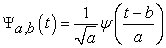(2)

Where a, b∈R, a>0 and R is the wavelet space. Parameters ‘a’ and ‘b’ are the scaling factor and the shifting factor, respectively, since choosing a prototype function as the mother wavelet should always satisfy the admissibility condition (Equation 3):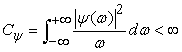(3)

Whereis the Fourier transform of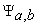. For any image with MxN pixels, discrete wavelet transform is calculated as equation (4).(4)

where I(x,y) is the pixel of image at x and y coordination, and g(x,y) is the wavelet wave. U and V indicate the wavelet space. We can use difference wavelet wave for this transform. Figure 7 exhibits the symlets wave. The symlets are nearly symmetrical wavelets proposed by Daubechies as modifications to the db family. The properties of the two wavelet families are similar [7, 8].

After wavelet transform, two type of coefficient is produced; approximation Coefficients Matrix and details coefficients matrix. Approximation coefficient shows low frequency content. It is what gives the images its identity. Details coefficient matrix depicts high frequency content. The high-frequency content, on the other hand, imparts flavor or nuance in the images.

The approximations are the high-scale, low-frequency components of the signal and the details are the low-scale, high-frequency components.

3.1. Continuous Wavelet Transform

First of all, each image is decomposed to red, green and blue images. Color of an image can carry much more information than gray level. Color is the most prominent feature of any image. Extracting color information from any image has many applications related to computer vision algorithms. This work shows in Figure 8. Then continuous wavelet transform is calculated: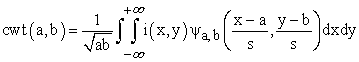(5)

Figure 9 shows 2D plot of soil image wavelet transform and Figure 10 shows 3D plot of this transform.

Continuous analysis is often easier to interpret, since its redundancy tends to reinforce the features and makes all information more visible. When the image is taken in discrete space (like this images), it is apparent that discrete wavelet transform could be more useful than continuous wavelet transform.

3.2. Discrete Wavelet Transform

Figure 11 presents approximation matrix and Figure 12 presents vertical, horizontal and diagonal details coefficient matrix of discrete wavelet transform of soil image. At approximation coefficient matrix, white and semi-white pixels depict high value of coefficient and it mains that edge and sharp changes are detected in image.

At details coefficient matrix, red and semi-red pixels depict high value of coefficient and it mains that edge and sharp changes are detected in image. So it is obvious that edge and sharp change in the image have a considerable wavelet coefficient and vice versa.

3.3. Discrete Energy of Each Coefficient

The wavelet energy provides information about the relative energy associated with different frequency bands presents in the image and its corresponding degree of importance. Energy of each coefficient can calculate with equation (6) and (7):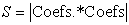(6)(7)
Figure 14. Percentage of energy for horizontal detail wavelet coefficient

where Coefs. is the wavelet transform coefficient and SC is the percentage of energy for etch wavelet coefficient. Figure 13 shows percentage of energy for approximation wavelet coefficient and Figure 14 shows percentage of energy for horizontal detail wavelet coefficient. As shown at these Figures, copper particles have a high energy percentage and background soil has a low energy. So estimation of copper density can be calculated with this percentage.

### 4. Copper Density Estimation

After calculation of wavelet coefficient energy for each pixel, it is compare with pre-selected threshold. In this paper, this value is set to 3.23x10-4 for copper particles. So if wavelet energy of each pixel is under this threshold; not any copper detected at there, and if wavelet energy of pixel is equal or over the threshold, it will be copper at that point.

### 5. Experimental Result

We capture color images from optical microscope in a Tabriz's copper mine. Images has a RGB format at a resolution of 550x710 pixels of 24 (8 bits for red, 8 bits for green and 8 bits for blue components). We develop and debug our vision algorithms using MATLAB software for execution on the computer vision. Proposed algorithm was tested for 100 images. Accuracy of this analyze is under ±7.60% error in copper area estimation. Symlets is picked out the best wavelet for mineral analyzing.

Hence, the grinding process of minerals can successfully be monitored on micrographic images by measuring the sizes and contents of each mineral grain. As applied in this paper, the processing of minerals based on light micrographic images of ore samples is quite cheap and fast as the images can be processed in a few seconds.

Our experiments confirmed that the methods proposed for segmenting minerals in micrographic images may also be applied for measuring and analyzing other textures in micrographic images.

The assignment of proper sample sizes for each mineral texture and the selection of correct samples of the grain may not be easy in some cases. Future work should focus on improving the performance of the system for those cases. The performance of the system can be improved by using high resolution color images taken by high quality camera systems. Additionally, hybrid methods utilizing advantages of different approaches should be applied to improve the system performance for the cases.

### 6. Conclusion

This paper has presented an overview of copper density estimation that uses computer vision and image processing technology. The work was undertaken in response to the perceived need in the mineral industry to save cost and increase innovation in this sector. In particular, it is targeted at reducing the cost of copper density estimation.

Our experimentation in this paper confirmed that we can successfully estimate copper area. We considered that this technique is sufficiently valid to use in mining industry. The computer vision allowed us to gain our first indication of the accuracy of the system in real operation condition.

The soil images are recorded by optical microscope and colored camera. Due to existence of these cameras for other purposes, suggested system had the advantage of being low cost and straightforward to install and maintain.

Accuracy of 92.4% in area estimation in soil images, in real area were obtained with wavelet transform of images. This transform had used symlets wave. Running test results in real area examined that our proposed algorithm method is accurate in all images.

### Acknowledgement

I would like to thank Dr. Sehhatkhah, chancellor of university collage of Roshdiyeh, for his support and leadership was invaluable in the production of this research. Thanks also go out to Mr. Daj, Vice chancellor of university collage of Roshdiyeh, for the great collaboration over the years.

### References

  Mining in Iran: www.en.wikipedia.org/Mining_in_IranIn article  Jurrus, E., Paiva, A., Watanabe, S., Anderson, J., Jones, B., et al. “Detection of neuron membranes in electron microscopy images using serial neural network architecture,” Med Image Anal, 14, 770-83, 2010.In article CrossRef PubMed  Torch, S., Usson, Y., Saxod, R., “Automated morphometric study of human peripheral nerves by image analysis”, Pathol Res Pract 185, 567-71, 2010.In article CrossRef  Zhao, X., Pan, Z., Wu, J., Zhou, G., Zeng, Y., “Automatic identification and morphometry of optic nerve fibers in electron microscopy images”, Comput Med Imaging Graph, 34, 179-84, 2010.In article CrossRef PubMed  MATLAB software help: www.mathworks.comIn article  Gonzalez, R.C., Woods, R.E., Digital Image Processing, Prentice Hall, 2007.In article  Haddad, S. C., Worden, R. H., Prior, D. J., and Smalley, P. C., Quartz cement in the Fontainebleau Sandstone, Paris Basin, France; crystallography and implications for mechanisms of cement growth: Journal of Sedimentary Research, v. 76, p. 244-256, 2006.In article CrossRef  Grelk, B., Review of literature and TEAM results, TEAM-project WP2, p. 40, 2005.In article